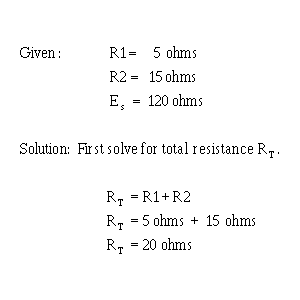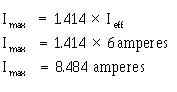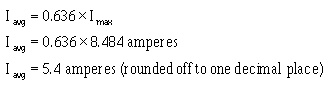Ohm's law in AC Circuits heating elements are examples of resistive elements. When an ac circuit contains only resistance, Ohm's Law, Kirchhoff's Law, and the various rules that apply to voltage, current, and power in a dc circuit also apply to the ac circuit.">Custom SearchOHM'S LAW IN AC CIRCUITS Many ac circuits contain resistance only. The rules for these circuits are the same rules that apply to dc circuits. Resistors, lamps, and heating elements are examples of resistive elements. When an ac circuit contains only resistance, Ohm's Law, Kirchhoff's Law, and the various rules that apply to voltage, current, and power in a dc circuit also apply to the ac circuit. The Ohm's Law formula for an ac circuit can be stated asRemember, unless otherwise stated, all ac voltage and current values are given as effective values. The formula for Ohm's Law can also be stated asThe important thing to keep in mind is: Do Not mix ac values. When you solve for effective values, all values you use in the formula must be effective values. Similarly, when you solve for average values, all values you use must be average values. This point should be clearer after you work the following problem: A series circuit consists of two resistors (R1 = 5 ohms and R2 = 15 ohms) and an alternating voltage source of 120 volts. What is Iavg?The alternating voltage is assumed to be an effective value (since it is not specified to be otherwise). Apply the Ohm's Law formula.The problem, however, asked for the average value of current (I avg). To convert the effective value of current to the average value of current, you must first determine the peak or maximum value of current, Imax.You can now find Iavg. Just substitute 8.484 amperes in the Iavg formula and solve for Iavg.Remember, you can use the Ohm's Law formulas to solve any purely resistive ac circuit problem. Use the formulas in the same manner as you would to solve a dc circuit problem. Q.41 A series circuit consists of three resistors (R1 = 10W, R2 = 20W, R3 = 15W) and an alternating voltage source of 100 volts. What is the effective value of current in the circuit? Q.42 If the alternating source in Q41 is changed to 200 volts peak-to-peak, what is Iavg? Q.43 If Eeff is 130 volts and Ieff is 3 amperes, what is the total resistance (RT) in the circuit?Integrated Publishing, Inc. - A (SDVOSB) Service Disabled Veteran Owned Small Business